Next: PSR B0042-73 in the Up: Black Holes in Binaries Previous: Binary Radiopulsars with Black

## Cyg X-1 as a Progenitor of BH+PSR Binaries

For massive binaries,Ā we assumed an initial mass ratio exponent, and obtained the total amount of both types of binary PSR with BH per Galaxy of2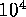, which is in agreement with independent estimates of Narayan et al. (1991). As we have shown in the previous section, roughly half of all binary BH+PSR systems in their evolutionary history must pass through the stage BH+II which can be observationally identified with Cyg X-1-like binaries. Using this correlation N(BH+II)-N(BH+PSR) we can obtain another independent estimate, which is free of selection effects, of the expected number of BH+PSR. In FigureĀ34 we plot the ratio of total calculated N(PSR+BH)+N(BH+PSR) to that of PSR, against calculated N(BH+II) for different combinations of parameters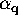,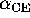,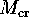,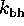and both isotropic and anisotropic BH collapse. The above estimate of number of bright BH+II binaries (similar to Cyg X-1), about 10 per Galaxy, yields a lower limit for the expected N(PSR+BH)0.3 per 700 single pulsars (Figure 34).

Ā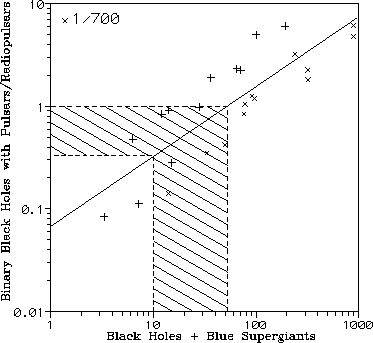Figure 34: Scenario machine calculation of galactic number of BH+PSR binaries against BH+II (Cyg X-1-like binaries), for different combinations of parameters of the evolutionary scenario. Crosses and pluses correspond to isotropic and anisotropic collapse to BH, respectively (Lipunov et al., 1994b).Ā

We note that when estimating the number of bright BHC binaries one misses dim X-ray BH+II systems with long orbital periods. From the parsity of PSR+BH binaries detected so far (an upper limit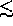1 per 700 known pulsars) we can infer an upper limit to the total galactic number of BH in pairs with blue supergiants100 per Galaxy (upper dashed line in FigureĀ34). These estimates mean that PSR+BH systems may be discovered in the near future.

Mike E. Prokhorov
Sat Feb 22 18:38:13 MSK 1997